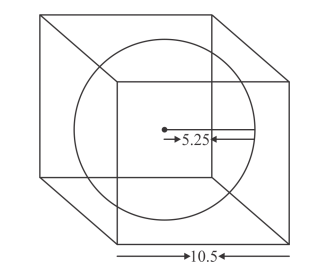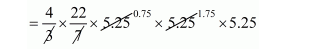# The largest sphere is carved out of a cube of side 10.5 cm.Question:

The largest sphere is carved out of a cube of side 10.5 cm. Find the volume of the sphere.

Solution:The side of cube a = 10.5 cm.

Since, a largest sphere is curved out of that cube

$r=\frac{a}{2}$

$r=\frac{10.5}{2} \mathrm{~cm}$

$r=5.25 \mathrm{~cm}$

The volume of sphere

$=\frac{4}{3} \pi(5.25)^{3}$$=22 \times 0.75 \times 1.75 \times 21$

$=606.375 \mathrm{~cm}^{3}$